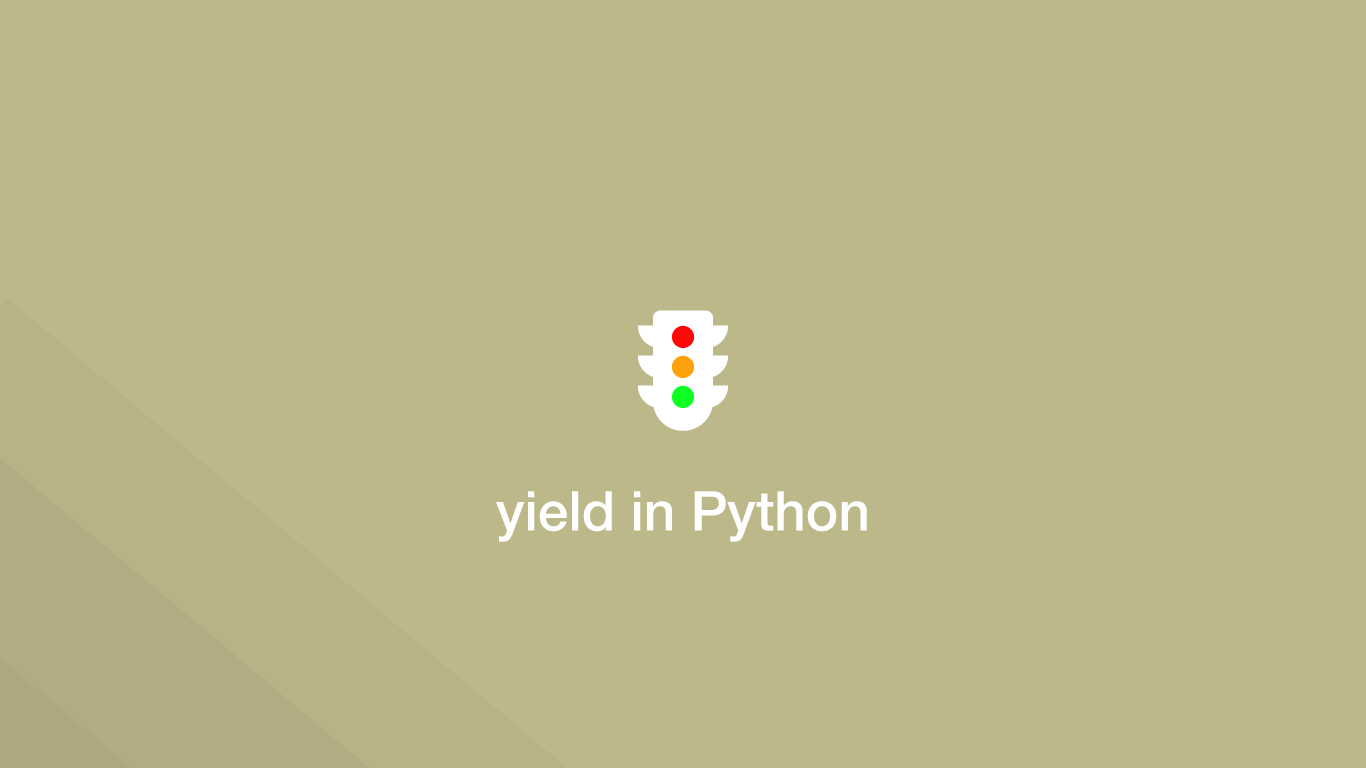# How to use yield Statements in PythonA `yield` statement in python is used to return a response back to the caller while leaving the function in a paused state that will resume once called again. This behaviour is different from the `return` statement, which ends the function completely with the next call having to start from the beginning of the function.

In this tutorial, we will learn how to use the Python yield statement to pause and resume the operation of a function.

## Using the yield Statement

`yield` can be used anywhere inside a function and can return a value or `None`. When the function object is called again using `next()` it will continue to run after the last yield statement. Let's create a function that will add different numbers to the input number on each call.

``````def generator(n):
yield n + 2
yield n + 4
yield n + 8

result = generator(1)

print(f'Result from first call is: {next(result)}')
print(f'Result from second call is: {next(result)}')
print(f'Result from third call is: {next(result)}')
``````
``````Result from first call is: 3
Result from second call is: 5
Result from third call is: 9
``````

## Yielding a Function with a for Loop

A function can provide data back in a staggered way by using `yield`. Let's create a `for` loop that will iterate over a function and print the result yielded back to it on each iteration.

``````def gen():
s = 'first string'
yield s
s = 'second string'
yield s
s = 'third string'
yield s

for i in gen():
print(i)
``````
``````first string
second string
third string
``````

## Using yield in Generator Functions

Generator functions are written like regular functions except they use `yield` to return data and resume from after the `yield` each time. This allows the function to be more abstract and allow some of the decision making to be done by the thing that is calling it.

``````def countWhile():
n = 1

while True:
yield n
n = n + 1

for num in countWhile():
if num > 10:
break
print(num)
``````
``````1
2
3
4
5
6
7
8
9
10
``````

In the above example, we are breaking the `while` loop in the `countWhile` generator function outside of the function in a `for` loop if the yielded value is above 10.

## Conclusion

You now know how to use the `yield` statement in Python and how it can be useful for situations where you don't want to have to run a function from the beginning each time.

#### Related Tutorials### How to Use if and else Statements in Python

August 31, 2020### How to Use Python List Comprehension

October 04, 2020### Python Assert Statement

September 30, 2020### How to Compare Strings in Python

September 20, 2020### How to use Python Try Except Block

June 14, 2021### Python Print Variable

September 16, 2021